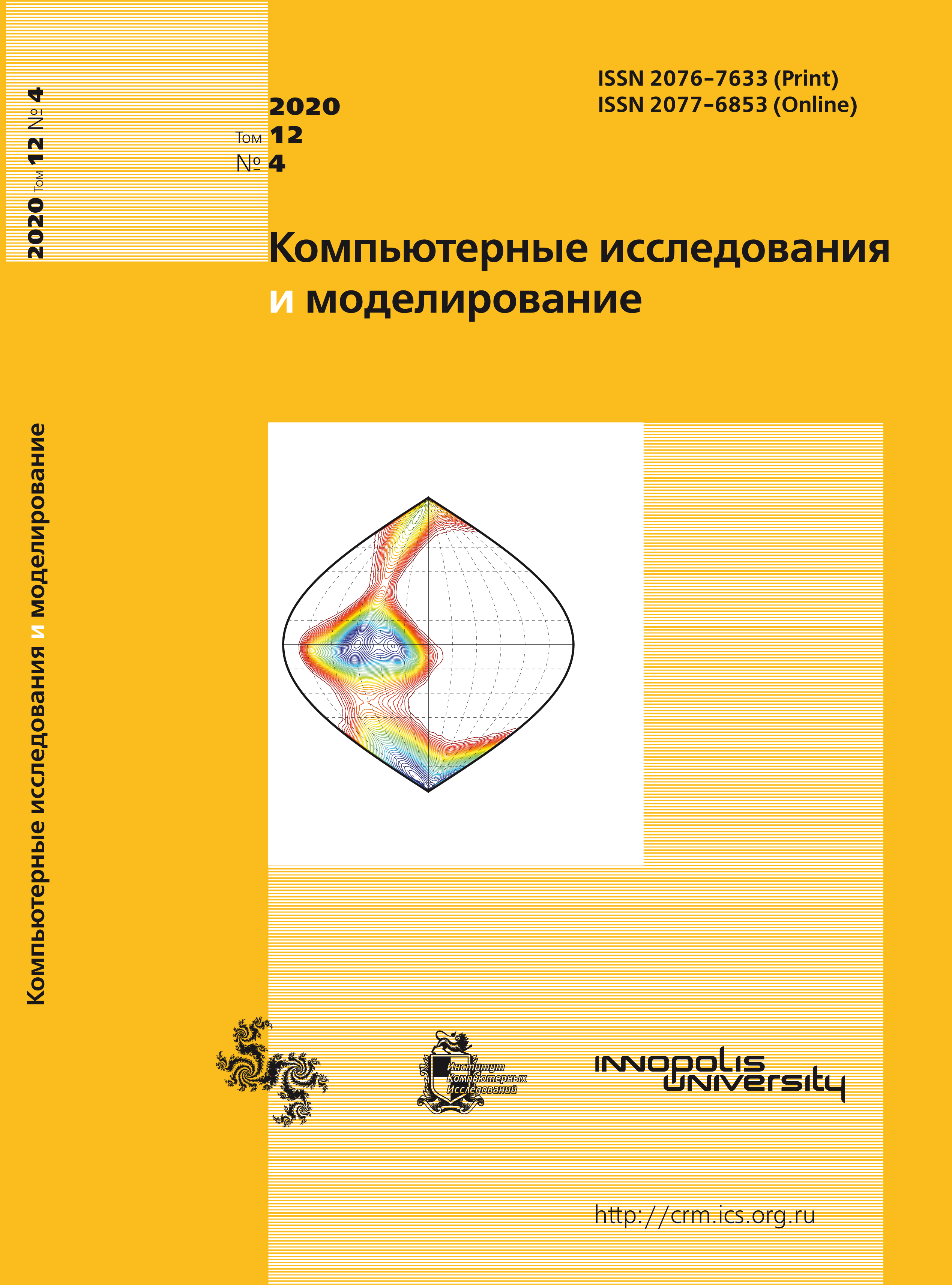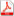Latest issue Issue 4, 2020 Vol. 12

# All issues

A method of constructing a predictive neural network model of a time seriespdf (2499K)

This article studies a method of constructing a predictive neural network model of a time series based on determining the composition of input variables, constructing a training sample and training itself using the back propagation method. Traditional methods of constructing predictive models of the time series are: the autoregressive model, the moving average model or the autoregressive model — the moving average allows us to approximate the time series by a linear dependence of the current value of the output variable on a number of its previous values. Such a limitation as linearity of dependence leads to significant errors in forecasting.

Mining Technologies using neural network modeling make it possible to approximate the time series by a nonlinear dependence. Moreover, the process of constructing of a neural network model (determining the composition of input variables, the number of layers and the number of neurons in the layers, choosing the activation functions of neurons, determining the optimal values of the neuron link weights) allows us to obtain a predictive model in the form of an analytical nonlinear dependence.

The determination of the composition of input variables of neural network models is one of the key points in the construction of neural network models in various application areas that affect its adequacy. The composition of the input variables is traditionally selected from some physical considerations or by the selection method. In this work it is proposed to use the behavior of the autocorrelation and private autocorrelation functions for the task of determining the composition of the input variables of the predictive neural network model of the time series.

In this work is proposed a method for determining the composition of input variables of neural network models for stationary and non-stationary time series, based on the construction and analysis of autocorrelation functions. Based on the proposed method in the Python programming environment are developed an algorithm and a program, determining the composition of the input variables of the predictive neural network model — the perceptron, as well as building the model itself. The proposed method was experimentally tested using the example of constructing a predictive neural network model of a time series that reflects energy consumption in different regions of the United States, openly published by PJM Interconnection LLC (PJM) — a regional network organization in the United States. This time series is non-stationary and is characterized by the presence of both a trend and seasonality. Prediction of the next values of the time series based on previous values and the constructed neural network model showed high approximation accuracy, which proves the effectiveness of the proposed method.

Keywords: time series, forecasting, neural network model, perceptron, trend, seasonality, stationary series, nonstationary series, autocorrelation function, private autocorrelation function, approximation accuracy
Citation in English: Emaletdinova L.Y., Mukhametzyanov Z.I., Kataseva D.V., Kabirova A.N. A method of constructing a predictive neural network model of a time series // Computer Research and Modeling, 2020, vol. 12, no. 4, pp. 737-756
Citation in English: Emaletdinova L.Y., Mukhametzyanov Z.I., Kataseva D.V., Kabirova A.N. A method of constructing a predictive neural network model of a time series // Computer Research and Modeling, 2020, vol. 12, no. 4, pp. 737-756
DOI: 10.20537/2076-7633-2020-12-4-737-756

Full-text version of the journal is also available on the web site of the scientific electronic library eLIBRARY.RU

The journal is included in the Russian Science Citation Index

The journal is included in the List of Russian peer-reviewed journals publishing the main research results of PhD and doctoral dissertations.

International Interdisciplinary Conference "Mathematics. Computing. Education"

The journal is included in the RSCI

Indexed in Scopus